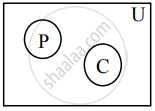# Represent the truth of the following statement by the Venn diagram. No circles are polygons. - Mathematics and Statistics

Sum

Represent the truth of the following statement by the Venn diagram.

No circles are polygons.

#### Solution

Let U : The set of all closed geometrical figures in plane.
P : The set of all polygons
C : The set of all circles.The above Venn diagram represents truth of the given statement, P ∩ C ≠ φ

Concept: Venn Diagrams
Is there an error in this question or solution?